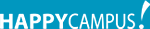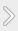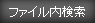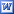#### 資料紹介

Determine the kinetic energy of the rocket firework by calculation. To calculate kinetic energy, 2 values are need. How high it went and how long it takes to reach zenith. Use a method call triangulation to determine how high the rocket firework went. It’s very simple technique. From 2 different points, each person checks the angle when the rocket firework reaches zenith, which is where the rocket firework pop in the air. Now, figure out how high the rocket firework went using 2 angles and the distance between those 2 angles. Then by using that value in kinematics formula, figure out the initial velocity. Already know the mass of rocket firework so, by using that value and initial velocity, calculate kinetic energy.

First of all, in my experiment, there were full of errors. Biggest part is when we measure the angle of how high the rocket firework went. Only way we could figure out the angle was to see by eyes and approximately measure angle by using protractor. By using this method, it’s impossible to figure out accurate result. However, this was only method I can really test. Also that day, it was windy and very bright (day time). It was very difficult to see where rocket firework pop. So we rely on smoke. As I said, that day there was wind so there is high possibility that smoke flow very quickly and provide wrong position. In conclusion, my experiment had so many problems. One another point was energy of “light”. I didn’t consider but there must have some energy transferred into “light”. If we have another chance, I would like to consider about energy of “light”. And if we do the experiment again, I want to choose windless nighttime. Because, if there less wind, the position of smoke doesn’t change and also in night time, I think we can see some light or flash when firework pop. If we can see that, we can record more accurate result.

### 資料の原本内容( この資料を購入すると、テキストデータがみえます。 )

*Aim；
Determine the kinetic energy of the rocket firework by calculation. To calculate kinetic energy, 2 values are need. How high it went and how long it takes to reach zenith. Use a method call triangulation to determine how high the rocket firework went. It's very simple technique. From 2 different points, each person checks the angle when the rocket firework reaches zenith, which is where the rocket firework pop in the air. Now, figure out how high the rocket fire..

## featherの資料(176)

•Relax
•Report image
•Simple and Stylish
•Stylish green
•Vision

### コメント追加

コメントを書込むには会員登録するか、すでに会員の方はログインしてください。